Courses

# Volumetric Analysis - Redox Reactions Chemistry Notes | EduRev

## Physical Chemistry

Created by: Asf Institute

## Chemistry : Volumetric Analysis - Redox Reactions Chemistry Notes | EduRev

The document Volumetric Analysis - Redox Reactions Chemistry Notes | EduRev is a part of the Chemistry Course Physical Chemistry.
All you need of Chemistry at this link: Chemistry

7.  VOLUMETRIC ANALYSIS

Now, we have developed enough platforms to understand the law of equivalents and volumetric analysis.

The volumetric analysis is an analytical method of estimating the concentration of a substance in a solution by adding exactly same number of equivalents of another substance present in the solution of known concentration .

This is the basic principle of titration. Volumetric analysis is also known as titrimetric analysis. The substance whose solution is employed to estimate the concentration of unknown solution is called titrant and the substance whose concentration is to be determined is called titrate.

The Volumetric Analysis is divided into following types:

1. Simple Titrations
2. Back Titrations
3. Double Titrations

7.1 SIMPLE TITRATIONS

The aim of simple titration is to find the concentration of an unknown solution with the help of the known concentration of another solution.

Let us take a solution of substance ‘A’ of unknown concentration. We are provided with solution of another substance ‘B’ whose concentration is known (N1). We take a certain known volume
(V2litres) of ‘A’ in a flask and start adding ‘B’ from burette to ‘A’ slowly till all the ‘A’ is consumed by ‘B’. This can be known with the aid of suitable indicator, which shows color change after the complete consumption of ‘A’. Let the volume of B consumed is V1 litre. According to the law of equivalents, the number of equivalents of ‘A’ would be equal to the number of equivalents of ‘B’.

N1V1 = N2 V2, where N2 is the concentration of ‘A’.

Thus using this equation, the value of N2 can be calculated.

There are four types of simple titrations, namely

(A) Acid-Base titrations

(B) Redox titrations

(C) Precipitation titrations

(D) Complexometric titrations

7.1.1    ACID-BASE TITRATIONS

In this type of titration , the concentration of an acid in a solution is estimated by adding a solution of standard base and vice-versa. The equivalence point is detected by adding a few drops of suitable indicator to the solution whose concentration is to be estimated. An acid-base indicator gives different colors with acids and bases . the choice of an indicator in a particular titration depends on the pH-range of the indicator and the pH change near the equivalence point. For example,

I. STRONG ACID –STRONG BASE TITRATION :

In the titration of HCl Vs NaOH, the equivalence point lies in pH-range of 4-10. Thus, methyl red ( pH-range 4.2 to 6.3 ) , methyl orange ( pH-range 3.1 to 4.4 )and phenolphthalein (pH-range 8.3 to 10) are suitable indicators.

II.WEAK ACID – STRONG BASE TITRATION :

In the titration of CH3COOH and NaOH , the equivalence point lies in pH-range of 7.5 to 10. Thus, phenolphthalein is the suitable indicator.

III.WEAK BASE – STRONG ACID TITRATION :

In the titration of NH4OH and HCl , the equivalence point lies in pH-range of 4 to 6.5. Thus, methyl orange and methyl red are the suitable indicators.

IV.WEAK ACID – WEAK BASE TITRATION :

In the titration of CH3COOH and NH4OH , , the equivalence point lies in

pH-range of 6.5 to 7.5 and the pH change is not sharp at the equivalence point. Thus, no simple indicator can be employed to detect the equivalence point.

7.1.2  REDOX TITRATIONS

In a redox titration, an oxidant is estimated by adding reductant or vice-versa. For example, Fe2+ ions can be estimated by titration against acidified KMnO4 solution when Fe2+ ions can be estimated by titration against acidified KMnO4 solution when Fe2+ ions are oxidized to Fe3+ ions and KMnO4 is reduced to Mn2+ in the presence of acidic medium. KMnO4 functions as self-indicator as its purple color is discharged at the equivalence point.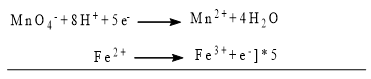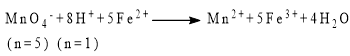In addition to acidified KMnO4 , acidified K2Cr2O7 can also be employed. Other redox titrations are iodimetry etc.

(a)  Iodimetry – This titration involves free iodine. Such direct estimation of iodine is called iodimetry. This involves the titration of iodine solution with known sodium thiosulphate solution, whose normality is N. Let the volume of sodium thiosulphate used be V litre.

I+ 2Na2S2O→ 2NaI + Na2S4O6

Equivalents of I2 = Equivalents of Na2S2O3 used = N x V

Moles of I2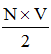Mass of free I2 in the solution =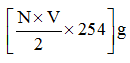(b) Iodometry – This is an indirect method  of estimation of iodine. An oxidizing agent is made to react with excess of solid KI . The oxidizing agent oxidizes I- to I2. This liberated iodine is then made to react with Na2S2O solution of normality N. Let the volume of thiosulphate solution required be V litre.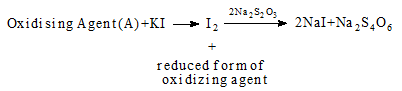Equivalents of ‘A’ = Equivalents of I2 = Equivalents of Na2S2Oused = N x V

Equivalents of I2 liberated from KI =  N x V

Equivalents of ‘A’ = N x V

Let the n-factor of ‘A’ in its reaction with KI be x, then

Mass of ‘A’ consumed =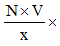MA( where Mis the molar mass of A )

7.1.3  PRECIPITATION TITRATIONS

In a titration of this kind, cations and anions combine to form a compound of very low solubility. Thus, a solid residue separates out. For example

AgNO3 + NaCl → AgCl↓(white) +NaNO3

BaCl2 + H2SO4 → BaSO4↓(white ) + 2HCl

7.1.4   COMPLEXOMETRIC TITRATIONS

In this type of titration, the titrate combines with the titrant to form complex salts . The complex salts may or may not be soluble. For example,

AgNO3 + 2KCN  →  K[Ag(CN)2] + KNO3

CuSO4 + 4NH4OH  →  [Cu(NH3)4]SO4 + 4H2O

7.2 BACK TITRATIONS

Let us assume that we have an impure solid substance ‘C’, weighing ‘w’ g and we are required to calculate the % purity of ‘C’ in the sample. We are also provided with two solutions ‘A’ and ‘B’, where the concentration of ‘B’ is known (N1) and that of ‘A’ is unknown. For the back titration to work, following conditions are to be satisfied (a) Compounds ‘A’, ‘B’ and ‘C’ should be such that ‘A’ and ‘B’ react with each other. (b) ‘A’ and pure ‘C’ also react with each other but the impurity present in ‘C’ does not react with ‘A’ . (c) Also the product of ‘A’ and ‘C’ should not react with ‘B’.

Now we take out certain volume of ‘A’ in flask (the equivalents of ‘A’ taken should be ≥ equivalents of pure ‘C’ in the sample ) and perform a simple titration using ‘B’ . Let us assume that the volume of ‘B’ used be Vlitre.

Equivalents of ‘B’ reacted with ‘A’ = N1V1

Equivalents of ‘A’ initially = N1V1

In another flask, we again take some volume of ‘A’ but now ‘C’ is added to this flask. Pure part of ‘C’ reacts with ‘A’ and excess of ‘A’ is back titrated with ‘B’. Let the volume of ‘B’ consumed is V2 litre .

Equivalents of ‘B’ reacted with excess of ‘A’ = N1V2

Equivalents of ‘A’ in excess = N1V

Equivalents of ‘A’ reacted with pure ‘C’ =( N1V- N1V)

Equivalents of pure ‘C’ = ( N1V- N1V)

Let the n-factor of ‘C’ in its reaction with ‘A’ be x, then the moles of pure ‘C’ = ( N1V- N1V)/x

Mass of pure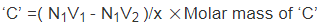% purity of ‘C’ =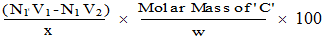7.3 DOUBLE TITRATIONS

The purpose of double titration is to determine the percentage composition of an alkali mixture or an acid mixture. In the present case, we will find the percentage composition of an alkali mixture. Let us consider a solid mixture of NaOH, Na2CO3 and some inert impurities, weighing ‘w’ g. We are required to find the % composition of this alkali mixture. We are also given an acid reagent (HCl) of known concentration M1 that can react with the alkali sample.

We first dissolve this mixture in water to make an alkaline solution and then we add two indicators, namely phenolphthalein and methyl orange to the solution. Now, we titrate this alkaline solution with standard HCl.

NaOH is a strong base while Na2CO3 is a weak base. So it is obvious that NaOH reacts first with HCl completely and Na2CO3 reacts only after complete NaOH is neutralized.

NaOH + HCl  → NaCl + H2O     ….. (i)

Once NaOH has reacted completely, then Na2CO3 starts reacting with HCl in two steps:

Na2CO3 + HCl  → NaHCO3+ NaCl ….. (ii)

NaHCO3 + HCl → NaCl + CO2 + H2O    ……. (iii)

It is clear that when we add HCl to the alkaline solution, alkali is neutralized and the pH of the solution decreases. Initially, the pH decrease would be rapid as strong base (NaOH) is neutralized completely. When Na2COis converted into NaHCO3 completely, the solution is still, weakly basic due to the presence of NaHCO3 (which is weaker as compared to Na2CO3). At this point, phenolphthalein changes color since it requires this weakly basic solution to show its color change. When HCl is further added, the pH again decreases and when all the NaHCO3 reacts to form NaCl, CO2 and H2O the solution becomes weakly acidic due to the presence of weak acid (H2CO3). At this point, methyl orange changes color as it requires this weakly acidic solution to show its color change.

Thus in general, phenolphthalein shows color change when the solution contains weakly basic NaHCO3 along with other neutral substances while methyl orange shows color change when solution contains weakly acidic H2CO3 along with other neutral substances .

Let the volume of HCl used up for the first and second reaction be V1 litre (this is volume of HCl used from the beginning of titration up to the point when phenolphthalein shows color change) and the volume of HCl required for the third reaction be V2 litre (this is the volume of HCl used from the point where phenolphthalein changes color upto the point when methyl orange shows color change. Then,

Moles of HCl consumed by NaHCO3 = Moles of NaHCO3 reacted = M1V2

Moles Of NaHCO3 formed from Na2CO3 = M1V2

Moles of Na2CO3 in the mixture = M1V2

Mass of Na2CO3 in the mixture = M1V2  x 106

% of Na2CO3 in the mixture = (M1Vx 106)/2 x 100

Moles of HCl used in the reaction (i) and (ii) = M1V1

Moles of HCl used in reaction (ii) = M1V2

Moles of HCl used in reaction (i) = (M1V- M1V2)

Moles of NaOH = (M1V- M1V2)

Mass of NaOH = (M1V- M1V2) x 40

% of NaOH in the mixture = [(M1V- M1V2)x40]/w  x 100

Here, we have determined the % composition of the mixture using mole concept, as the balanced reactions were available. If we were to solve this by equivalent concept, then the procedure adopted would be

NaOH   +    HCl  →    NaCl    +   H2O(i)

Na2CO3  +  HCl    →   NaHCO3 + NaCl  (ii)

(n=1)  (n=1)   (n=1)

Phenolphthalein shows end point after reaction (ii)

NaHCO3   +   HCl →  NaCl   +  CO2  +  H2O

(n=1)         (n=1)

And methyl orange shows end point after reaction (iii).

At phenolphthalein end point,

(equivalents of HCl )1 =equivalents of NaOH +equivalents of Na2CO3 (n=1)……..(iv)

= M1V1

At methyl orange end point,

(equivalents of HCl )2 = equivalents of NaHCOreacted ( n=1 )

= equivalents of NaHCOproduced ( n=1 ) = equivalents of Na2CO3 ( n=1 )

M1V= Equivalents of Na2CO3 ( n=1 ) = ½ equivalents of Na2CO3 ( n=2 )  …… (v)

Subtracting the equation (v) from (iv),]

Equivalents of NaOH = (M1V- M1V2)

Moles of NaOH = (M1V- M1V2)          (Since n-factor of NaOH is 1 )

% of NaOH in the mixture = [ (M1V- M1V2) x 40 ]/w  x 100

Equivalents of Na2CO3 ( n=1 ) = M1V2

And moles of Na2CO3 = M1V2

% of Na2CO3 in the mixture =( M1Vx106 )/w  x 100

In the above case, we have taken alkali mixture of NaOH and Na2CO3. But other alkali mixtures can also be taken. For example,

(i) If a mixture of NaOH and NaHCO3 has been taken

(a) Equivalents of NaOH = Equivalents of HCl required upto phenolphthalein end point

(b) Equivalents of NaHCO3 = Equivalents of HCl required from phenolphthalein end point to methyl orange end point.

(ii) If a mixture of Na2CO3 and NaHCO3 has been taken

(a)  ½ equivalents of Na2CO3 ( n=2 ) = Equivalents of HCl required upto phenolphthalein end point.

(b)  ½ equivalents of  Na2CO3 ( n=2 ) = Equivalents of NaHCO3 initially ( n=1 ).

= Equivalents of HCl required from phenolphthalein end point to methyl orange end point.

(iii) If a mixture of NaOH, Na2CO3 and NaHCO3 has been taken

(a)  Equivalents of NaOH + ½ Equivalents of Na2CO3 (n=2)

= Equivalents of HCl required up to phenolphthalein end point.

(b)  ½ equivalents of  Na2CO3 ( n=2 ) = Equivalents of NaHCO3 initially ( n=1 ).

=Equivalents of HCl required from phenolphthalein end point to methyl orange end point.

8.   VOLUME STRENGTH OF H2O2 SOLUTION

When a solution of H2O2 is labeled as ‘x volumes’ , it means that  1 volume ( 1ml or 1 L ) of H2O2 solution would liberate x volumes ( x ml or x L) of O2 at STP on complete decomposition.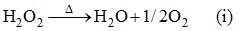If a H2Osolution  (acting as reducing agent ) has normality N and it is to be reacted with KMnO4 solution ( acting as oxidizing agent ), we can say that there are N equivalents of H2O2 present in 1 L of this H2Osolution. 1 ml of H2O`of this solution would contain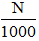equivalents.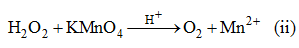Moles of H2Oin 1 ml of this solution =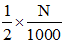[from equation (ii) ] When these many moles of H2O2 in 1 ml of solution are allowed to decompose according to the reaction,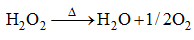the volume of O2 released ( in ml ) by them at STP will give the volume trength of H2O2 solution. Moles of O2 given by 1 ml of this solution =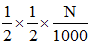[from eq. (i)]

Volume of O2 at STP given by 1 ml of this solution =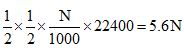Volume strength of H2O2 = 5.6 x Normality of H2O2

9 . PERCENTAGE LABELING OF OLEUM

Oleum contains H2SO4 and SO3 only. When Oleum is diluted ( by adding water ) , SO3 reacts with H2O to form H2SO4 , thus increasing the mass of the solution.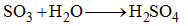The total mass of H2SOobtained by diluting 100 g of oleum sample with required amount of water, is equal to the percentage labeling of oleum.

Percentage labeling of oleum = Total mass of H2SO4 present in oleum after dilution.

=  mass of H2SOinitially present + mass of H2SO produced on dilution.

If we have a sample of oleum labeled as 109%, this means that 100g of oleum on dilution gives 109g of H2SO4. Let us calculate the composition of oleum, which is labeled as 109%.

Let the mass of SO3 in the sample be x g, then the mass of H2SO4 would be ( 100 – x)g. On dilution,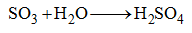Moles of SO3 in oleum = x/80 = Moles of H2SOformed on dilution.

Mass of H2SOformed on dilution =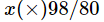Total mass of H2SO4 present in oleum after dilution =+ (100-x) = 109. Thus, x=40 . Thus, oleum contained 40% SO3 and 60% H2SO4.

Atlernatively, let the mass of oleum sample be 100g, which on dilution becomes 109g. This implies that 9g of H2O was added.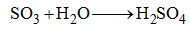Moles of H2O added = 9/18 = Moles of SO3 present in oleum sample.

Mass of SO3 in oleum = (9/18) x 80 = 40g.

Thus oleum sample contained 40% SO3 of and 60% of H2SO4

10. MODIFICATIONS IN LAW OF EQUIVALENCE

There are certain modifications required in order to use law of equivalence. These modification are

(i) The equivalents of a substance produced and reacted may not necessarily be same . If the n-

factor of the substance, in the reaction in which it is produced were different than the n-

factorof the same substance,when it is reacting, then the equivalent of the substance

produced and reacted would be different.

(ii) The equivalents of the same substances can be added or subtracted only when they are having the same n-factor.

(iii) In a reaction, the equivalents of oxidizing agents would always be equal to the equivalents of reducing agents, irrespective of the number of agents used in the reaction.

Offer running on EduRev: Apply code STAYHOME200 to get INR 200 off on our premium plan EduRev Infinity!

## Physical Chemistry

77 videos|83 docs|32 tests

,

,

,

,

,

,

,

,

,

,

,

,

,

,

,

,

,

,

,

,

,

;